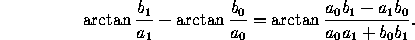Next: 4.3 Concurrence and Collinearity
Up: 4 Lines
Previous: 4.1 Distances

# 4.2 Angles

The angle between two lines ax+by+c=0 and ax+by+c=0 isIn particular, the two lines are parallel when ab=ab, and perpendicular when aa=-bb.

The angle between two lines of slopes mand mis

arctan((m-m)/(1+mm)).

In particular, the two lines are parallel when m=mand perpendicular when mm=-1.

Next: 4.3 Concurrence and Collinearity
Up: 4 Lines
Previous: 4.1 DistancesThe Geometry Center Home Page

Silvio Levy
Wed Oct 4 16:41:25 PDT 1995

This document is excerpted from the 30th Edition of the CRC Standard Mathematical Tables and Formulas (CRC Press). Unauthorized duplication is forbidden.